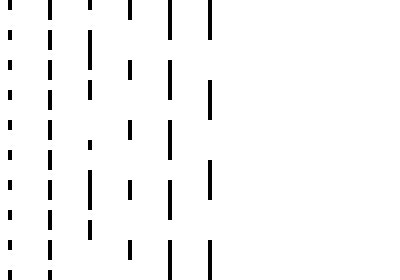# Styles and colors#

## Colors#

The `Canvas` has two color attributes, one for the strokes, and one for the surfaces. You can also change the global transparency.

• `stroke_style`: (valid HTML color)

The color for rectangles and paths stroke. Default to ‘black’.

• `fill_style`: (valid HTML color)

The color for filling rectangles and paths. Default to ‘black’.

• `global_alpha`: (float)

Transparency level. Default to 1.0.

```from ipycanvas import Canvas

canvas = Canvas(width=200, height=200)

canvas.fill_style = "red"
canvas.stroke_style = "blue"

canvas.fill_rect(25, 25, 100, 100)
canvas.clear_rect(45, 45, 60, 60)
canvas.stroke_rect(50, 50, 50, 50)

canvas
```There are two canvas methods that allow you to create color gradients (either linear or radial) that you can use as a `fill_style` or `stroke_style`.

• `create_linear_gradient(x0, y0, x1, y1, color_stops)`:

Create a `LinearGradient` object given the start point `(x0, y0)`, end point `(x1, y1)`, and color stops.

• `create_radial_gradient(x0, y0, r0, x1, y1, r1, color_stops)`:

Create a `RadialGradient` object given the start circle `(x0, y0, r0)`, end circle `(x1, y1, r1)`, and color stops.

You need to provide the start point and end point of your gradient (those coordinates are global to the entire canvas, it’s not per-shape). The color stops is a list of tuple `(offset, color)`, `offset` being a number between 0 and 1, inclusive, representing the position of the color stop. 0 represents the start of the gradient and 1 represents the end.

```from ipycanvas import Canvas

canvas = Canvas(width=700, height=50)

0,
0,  # Start position (x0, y0)
700,
0,  # End position (x1, y1)
# List of color stops
[
(0, "red"),
(1 / 6, "orange"),
(2 / 6, "yellow"),
(3 / 6, "green"),
(4 / 6, "blue"),
(5 / 6, "#4B0082"),
(1, "violet"),
],
)

canvas.fill_rect(0, 0, 700, 50)

canvas
```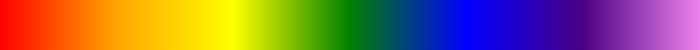```from ipycanvas import Canvas

canvas = Canvas(width=570, height=200)

238,
50,
10,  # Start circle (x0, y0, r0)
238,
50,
300,  # End circle (x1, y1, r1)
[
(0, "#8ED6FF"),
(1, "#004CB3"),
],
)

canvas.fill_rect(0, 0, 570, 200)

canvas
```## Patterns#

ipycanvas provides a mean to easily create patterns. You can create a pattern from an `ipywidgets.Image` widget, from a `Canvas` or a `MultiCanvas`, the created pattern can then be used as a `fill_style` or `stroke_style`.

• `create_pattern(image, repetition='repeat')`:

Create a `Pattern` object given the image source (either an `ipywidgets.Image`, a `Canvas` or a `MultiCanvas`), and the `repetition` which is a string indicating how to repeat the pattern’s image, can be “repeat” (both directions), “repeat-x” (horizontal only), “repeat-y” (vertical only), “no-repeat” (neither direction).

First create your pattern source or load it from an Image file with `ipywidgets.Image`:

```from math import pi

from ipycanvas import Canvas

pattern_source = Canvas(width=50, height=50)

pattern_source.fill_style = "#fec"
pattern_source.fill_rect(0, 0, 50, 50)
pattern_source.stroke_arc(0, 0, 50, 0, 0.5 * pi)

pattern_source
```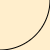Then use it as a `fill_style` or `stroke_style`:

```canvas = Canvas(width=200, height=200)

pattern = canvas.create_pattern(pattern_source)

canvas.fill_style = pattern
canvas.fill_rect(0, 0, canvas.width, canvas.height)

canvas
```## Filters#

You can apply filters such as blurring and grayscaling to your drawings using the `filter` property. This property is similar to the filter CSS property and accepts the same values.

The `filter` property is a `string` with the following syntax:

```canvas.filter = "<filter-function1> [<filter-function2>] [<filter-functionN>]"
canvas.filter = "none"
```

The following filter functions are supported:

• `url()`:

A CSS url(). Takes an IRI pointing to an SVG filter element, which may be embedded in an external XML file.

• `blur()`:

A CSS <length>. Applies a Gaussian blur to the drawing. It defines the value of the standard deviation to the Gaussian function, i.e., how many pixels on the screen blend into each other; thus, a larger value will create more blur. A value of 0 leaves the input unchanged.

• `brightness()`:

A CSS <percentage>. Applies a linear multiplier to the drawing, making it appear brighter or darker. A value under 100% darkens the image, while a value over 100% brightens it. A value of 0% will create an image that is completely black, while a value of 100% leaves the input unchanged.

• `contrast()`:

A CSS <percentage>. Adjusts the contrast of the drawing. A value of 0% will create a drawing that is completely black. A value of 100% leaves the drawing unchanged.

• `drop-shadow()`:

Applies a drop shadow effect to the drawing. A drop shadow is effectively a blurred, offset version of the drawing’s alpha mask drawn in a particular color, composited below the drawing. This function takes up to five arguments:

• <offset-x>: Specifies the horizontal distance of the shadow.

• <offset-y>: Specifies the vertical distance of the shadow.

• <blur-radius>: The larger this value, the bigger the blur, so the shadow becomes bigger and lighter. Negative values are not allowed.

• <color>: The shadow color.

• `grayscale()`:

A CSS <percentage>. Converts the drawing to grayscale. A value of 100% is completely grayscale. A value of 0% leaves the drawing unchanged.

• `hue-rotate()`:

A CSS <angle>. Applies a hue rotation on the drawing. A value of 0deg leaves the input unchanged.

• `invert()`:

A CSS <percentage>. Inverts the drawing. A value of 100% means complete inversion. A value of 0% leaves the drawing unchanged.

• `opacity()`:

A CSS <percentage>. Applies transparency to the drawing. A value of 0% means completely transparent. A value of 100% leaves the drawing unchanged.

• `saturate()`:

A CSS <percentage>. Saturates the drawing. A value of 0% means completely un-saturated. A value of 100% leaves the drawing unchanged.

• `sepia()`:

A CSS <percentage>. Converts the drawing to sepia. A value of 100% means completely sepia. A value of 0% leaves the drawing unchanged.

• `none`:

No filter is applied. Initial value.

```from ipycanvas import Canvas

canvas = Canvas(width=400, height=300)

canvas.fill_style = "green"
canvas.filter = "blur(1px) contrast(1.4) drop-shadow(-9px 9px 3px #e81)"
canvas.font = "48px serif"
canvas.fill_text("Hello world!", 20, 150)

canvas
```Warning

Applying a filter can be slow, especially if you draw many shapes.

Danger

This property is not supported by Safari and Internet Explorer

## RoughCanvas#

ipycanvas provides a special `Canvas` class which will automatically give a hand-drawn style to your drawings: see the Rough Canvas section.

You can easily draw shadows by tweaking the following attributes:

• `shadow_offset_x`: (float)

Indicates the horizontal distance the shadow should extend from the object. This value isn’t affected by the transformation matrix. The default is `0`.

• `shadow_offset_y`: (float)

Indicates the vertical distance the shadow should extend from the object. This value isn’t affected by the transformation matrix. The default is `0`.

• `shadow_blur`: (float)

Indicates the size of the blurring effect; this value doesn’t correspond to a number of pixels and is not affected by the current transformation matrix. The default value is `0`.

• `shadow_color`: (valid HTML color)

A standard CSS color value indicating the color of the shadow effect; by default, it is fully-transparent black: `'rgba(0, 0, 0, 0)'`.

```from ipycanvas import Canvas

canvas = Canvas(width=200, height=200)

canvas.fill_rect(25, 25, 100, 100)
canvas.clear_rect(45, 45, 60, 60)

canvas.stroke_rect(50, 50, 50, 50)

canvas
```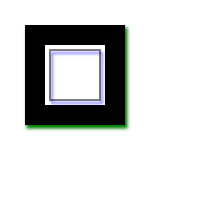## Lines styles#

You can change the following `Canvas` attributes in order to change the lines styling:

• `line_width`: (float)

Sets the width of lines drawn in the future, must be a positive number. Default to 1.0.

• `line_cap`: (str)

Sets the appearance of the ends of lines, possible values are ‘butt’, ‘round’ and ‘square’. Default to ‘butt’.

• `line_join`: (str)

Sets the appearance of the “corners” where lines meet, possible values are ‘round’, ‘bevel’ and ‘miter’. Default to ‘miter’

• `miter_limit`: (float)

Establishes a limit on the miter when two lines join at a sharp angle, to let you control how thick the junction becomes. Default to 10..

• `get_line_dash()`:

Return the current line dash pattern array containing an even number of non-negative numbers.

• `set_line_dash(segments)`:

Set the current line dash pattern.

• `line_dash_offset`: (float)

Specifies where to start a dash array on a line. Default is 0..

### Line width#

Sets the width of lines drawn in the future.

```from ipycanvas import Canvas

canvas = Canvas(width=400, height=280)
canvas.scale(2)

for i in range(10):
width = 1 + i
x = 5 + i * 20
canvas.line_width = width

canvas.fill_text(str(width), x - 5, 15)

canvas.begin_path()
canvas.move_to(x, 20)
canvas.line_to(x, 140)
canvas.stroke()
canvas
```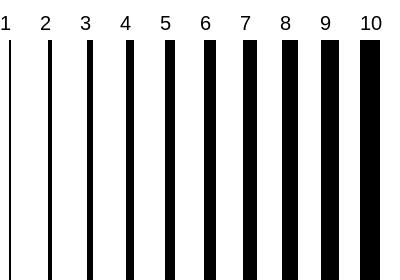### Line cap#

Sets the appearance of the ends of lines.

```from ipycanvas import Canvas

canvas = Canvas(width=320, height=360)

# Possible line_cap values
line_caps = ["butt", "round", "square"]

canvas.scale(2)

# Draw guides
canvas.stroke_style = "#09f"
canvas.begin_path()
canvas.move_to(10, 30)
canvas.line_to(140, 30)
canvas.move_to(10, 140)
canvas.line_to(140, 140)
canvas.stroke()

# Draw lines
canvas.stroke_style = "black"
canvas.font = "15px serif"

for i in range(len(line_caps)):
line_cap = line_caps[i]
x = 25 + i * 50

canvas.fill_text(line_cap, x - 15, 15)
canvas.line_width = 15
canvas.line_cap = line_cap
canvas.begin_path()
canvas.move_to(x, 30)
canvas.line_to(x, 140)
canvas.stroke()

canvas
```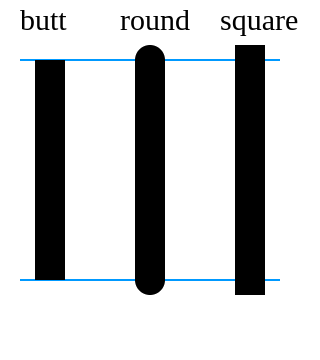### Line join#

Sets the appearance of the “corners” where lines meet.

```from ipycanvas import Canvas

canvas = Canvas(width=320, height=360)

# Possible line_join values
line_joins = ["round", "bevel", "miter"]

min_y = 40
max_y = 80
spacing = 45

canvas.line_width = 10
canvas.scale(2)
for i in range(len(line_joins)):
line_join = line_joins[i]

y1 = min_y + i * spacing
y2 = max_y + i * spacing

canvas.line_join = line_join

canvas.fill_text(line_join, 60, y1 - 10)

canvas.begin_path()
canvas.move_to(-5, y1)
canvas.line_to(35, y2)
canvas.line_to(75, y1)
canvas.line_to(115, y2)
canvas.line_to(155, y1)
canvas.stroke()

canvas
```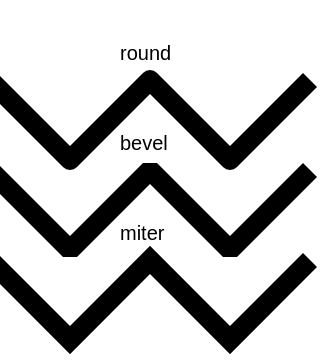### Line dash#

Sets the current line dash pattern.

```from ipycanvas import Canvas

canvas = Canvas(width=400, height=280)
canvas.scale(2)

line_dashes = [[5, 10], [10, 5], [5, 10, 20], [10, 20], [20, 10], [20, 20]]

canvas.line_width = 2

for i in range(len(line_dashes)):
x = 5 + i * 20

canvas.set_line_dash(line_dashes[i])
canvas.begin_path()
canvas.move_to(x, 0)
canvas.line_to(x, 140)
canvas.stroke()
canvas
```